# A Simple Ratio Test Example

Previous: The Ratio Test Flowchart

Next: Ratio Test Example with an Exponent

## Question

Using only the ratio test, determine whether or not the recursive sequenceconverges or diverges.

## Complete Solution

Applying the ratio test yieldsBut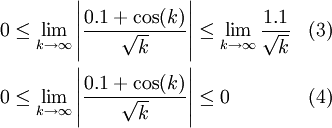Therefore,Since the limit equals, the ratio test tells us that the series converges.

## Explanation of Each Step

### Step (1)

To apply the ratio test, we must evaluate the limit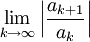In our problem, we can use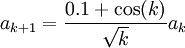and substitute this into our limit.

### Step (2)

In Step (2), we only cancel thein the numerator and denominator.

### Step (3)

First observe that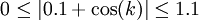Dividing everything by the square root of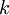we obtain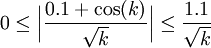### Step (4)

In Step (4) we only evaluate the limit: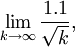which equals zero because the numerator is a constant and the denominator goes to infinity.

### Step (5)

In Step (5) we apply the Squeeze Theorem.

## Potential Challenge Areas

### Getting Started

Because the question asks us to apply the ratio test, we know that we will start our solution by using the formula### Recursive Formula

Most problems involving convergence tests don't involve recursive formulas. But with the ratio test, we applyand use the given recursion equation for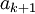. In our case, our recursion equation iswhich we substitute into the numerator, allowing us to cancel thein the numerator and denominator. This trick is a bit harder to apply for the other convergence tests.

Previous: The Ratio Test Flowchart

Next: Ratio Test Example with an Exponent